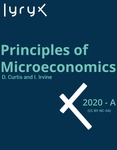## Open Access Textbooks#### Title

Principles of Microeconomics

#### Contributors

Doug Curtis - Author / Ian Irvine - Author

#### Description

Principles of Microeconomics is an adaptation of the textbook/ Microeconomics: Markets/ Methods/ and Models by D. Curtis and I. Irvine/ which provides concise yet complete coverage of introductory microeconomic theory/ application and policy in a Canadian and global environment. This adaptation employs methods that use equations sparingly and do not utilize calculus. The key issues in most chapters are analyzed by introducing a numerical example or case study at the outset. Students are introduced immediately to the practice of taking a data set/ examining it numerically/ plotting it/ and again analyzing the material in that form. The end-of-chapter problems involve numerical and graphical analysis/ and a small number of problems in each chapter involve solving simple linear equations (intersecting straight lines). However/ a sufficient number of questions is provided for the student to test understanding of the material without working through that subset of questions. This textbook is intended for a one-semester course/ and can be used in a two-semester sequence with the companion textbook/ Principles of Macroeconomics. The three introductory chapters are common to both textbooks.

#### Subject 1

Social Sciences - Economics

Lyryx

#### Resources

Open Textbook Library